Function Repository Resource:

# TrianglePointData

Given a 2D triangle and a point, get the specified data

Contributed by: Minh Trinh Xuan
 ResourceFunction["TrianglePointData"][{a,b,c},p,property] Given triangle with coordinates {a,b,c} and point p, return property (see details). ResourceFunction["TrianglePointData"][Triangle[tri],Point[p],property] Given triangle tri and point p, return property (see details). ResourceFunction["TrianglePointData"][Triangle[tri],Point[p],"Pedal"] Given triangle tri and point p, return the triangle formed by the altitudes.

## Details

Given triangle ABC and a point P (not on edge), then

 Trilinear distance from P to triangle edges Barycentric (u,v,w) = areas (PBC,APC,ABP) normalized so that u+v+w=1 InverseInCircum Point X satisfies OX × OM = R2 where O is the circumcenter Complement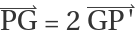, where G is the triangle centroid Anticomplement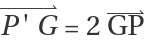, where G is the triangle centroid Isogonal Reflect cevians by angle bisectors Isotomic Reflect cevians by midpoints Cyclocevian Draw circumcircle of cevian points, use other intersections Antigonal The isogonal conjugate of the inverse-in-circumcircle of the isogonal conjugate of P.("Pairs of Points: Antigonal, Isogonal, and Inverse," Mathematics Magazine 65 (1992) 339-347) Synagonal The antigonal image of the anticomplement of P. (following Hyacinthos #9881) Cevian The triangle made by the cevians Anticevian The triangle with ABC as the cevian triangle Pedal The triangle made by the altitudes Antipedal The triangle with ABC as the pedal triangle

## Examples

### Basic Examples (2)

Given a triangle and point p, find the antipedal triangle:

 In:=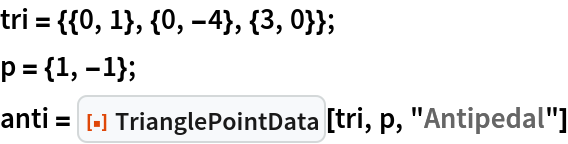Out=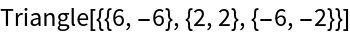Edges perpendicular to lines from p to the triangle vertices give the antipedal triangle:

 In:=Out=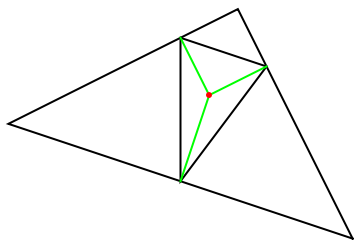### Scope (6)

Show the trilinear:

 In:=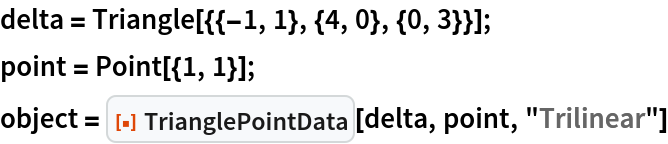Out=Show the barycentric:

 In:=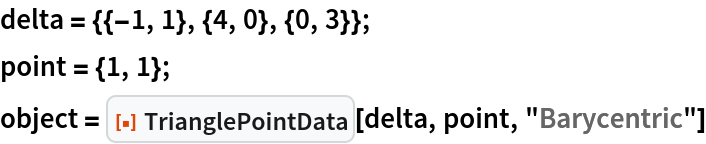Out=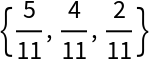Show a set of derived points:

 In:=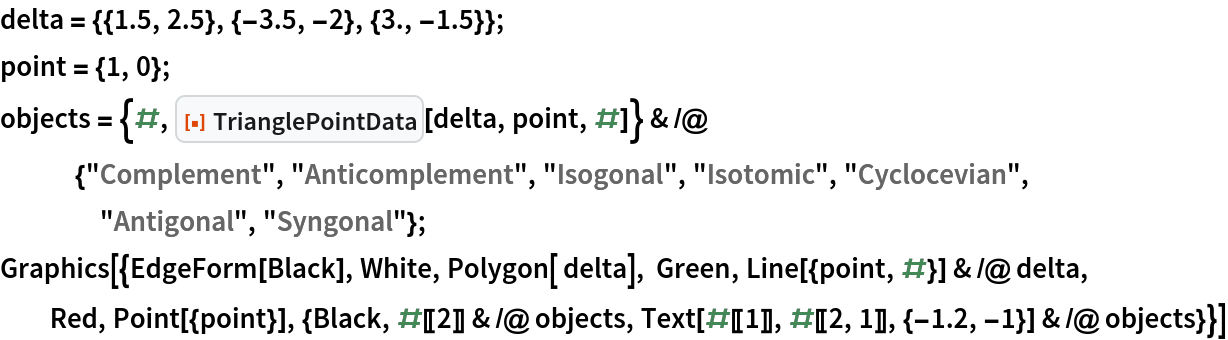Out=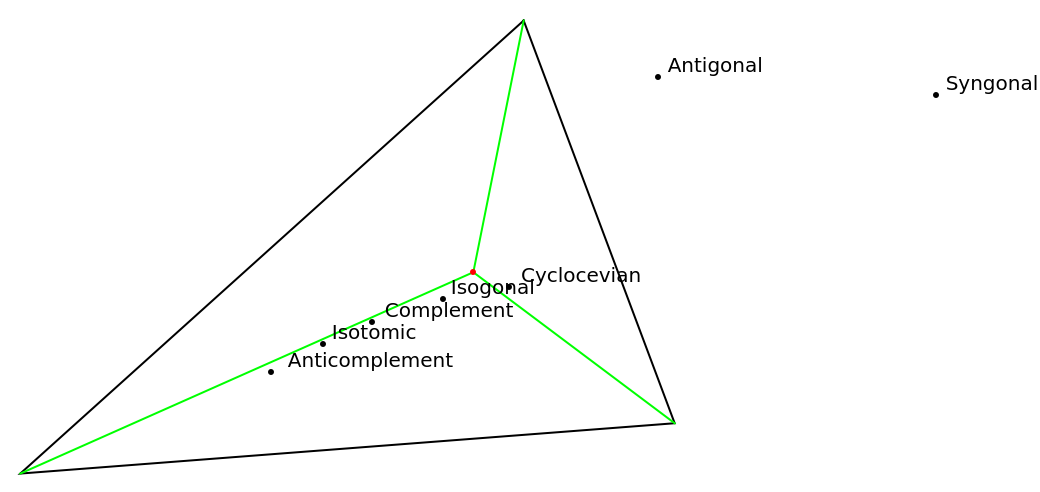Show the cevian triangle:

 In:=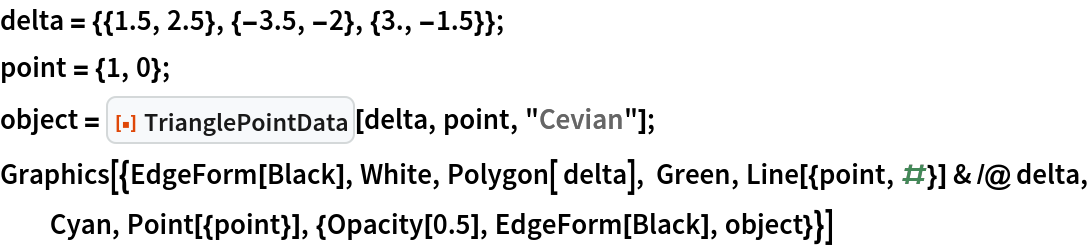Out=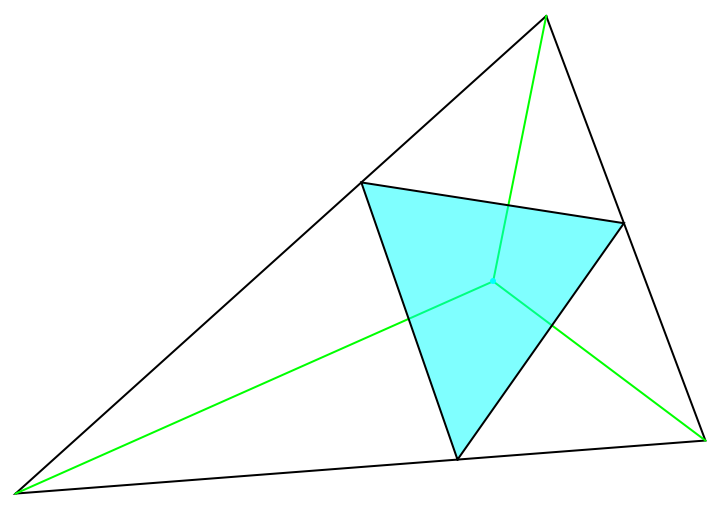Show the anticevian triangle:

 In:=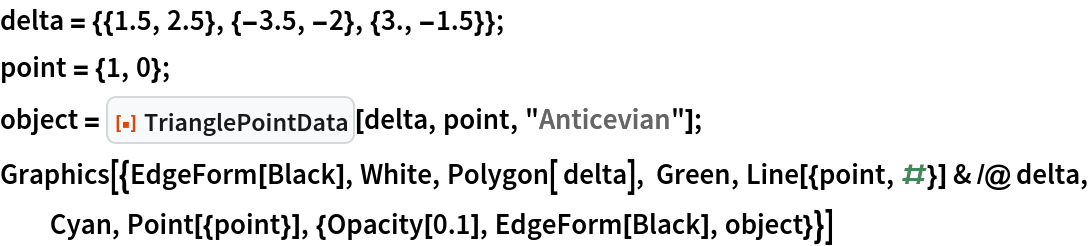Out=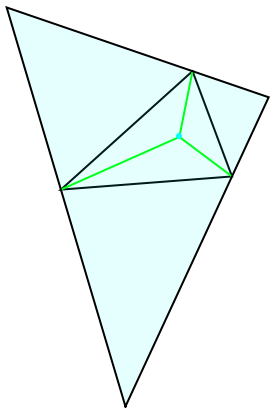Show the pedal triangle:

 In:=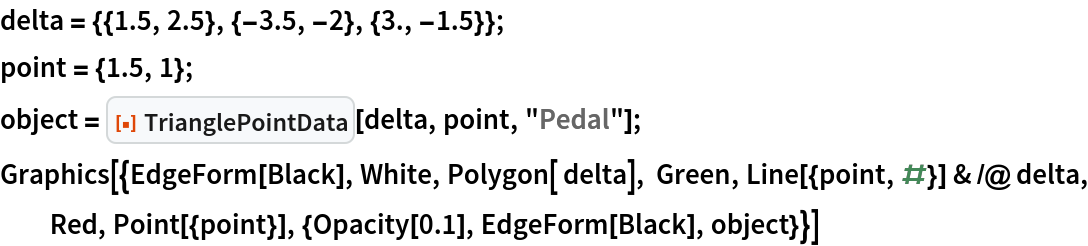Out=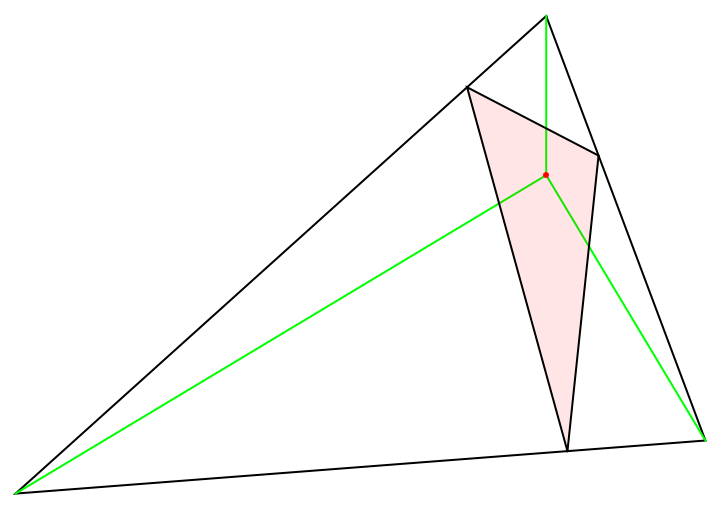### Neat Examples (1)

Construct the pedal triangle of a point:

 In:=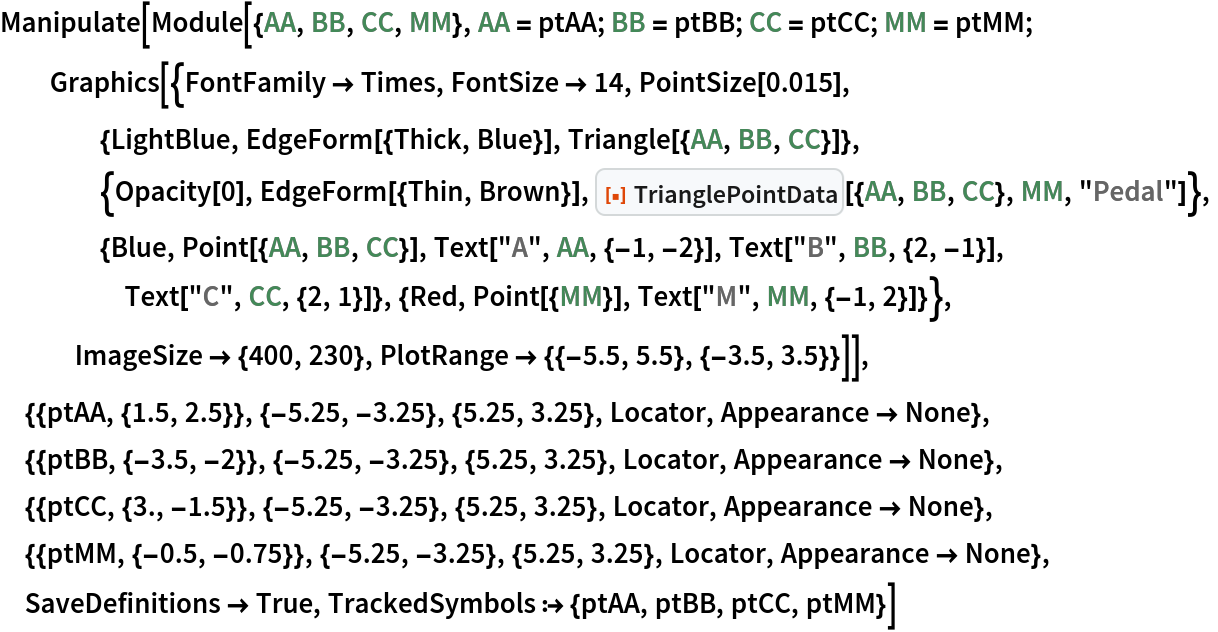Out=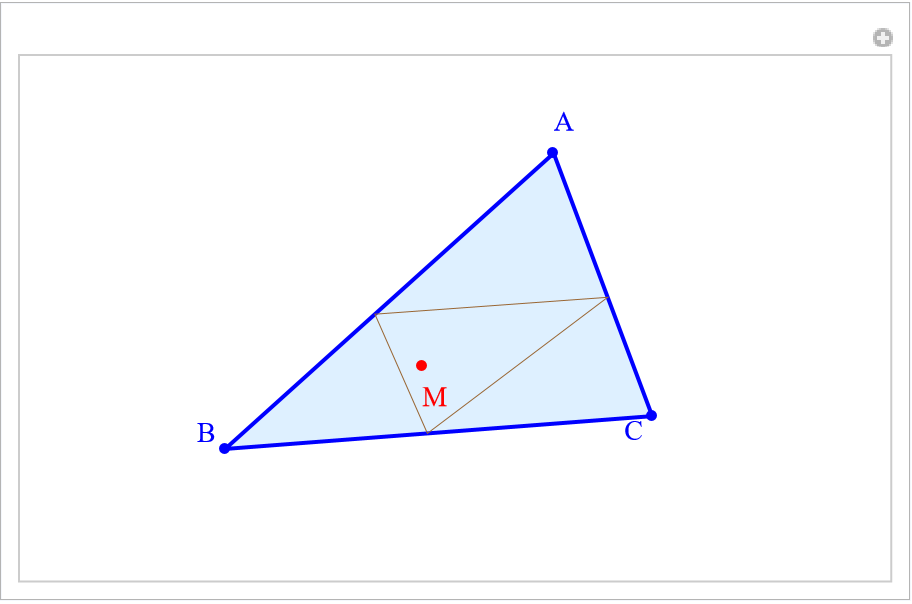## Version History

• 1.0.0 – 07 November 2022